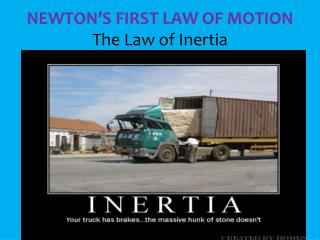DownloadDownload PresentationNEWTON’S FIRST LAW OF MOTION The Law of Inertia

# NEWTON’S FIRST LAW OF MOTION The Law of Inertia

Download Presentation## NEWTON’S FIRST LAW OF MOTION The Law of Inertia

- - - - - - - - - - - - - - - - - - - - - - - - - - - E N D - - - - - - - - - - - - - - - - - - - - - - - - - - -
##### Presentation Transcript

1. NEWTON’S FIRST LAW OF MOTIONThe Law of Inertia

2. The first law says that an object at rest tends to stay at rest, and an object in motion tends to stay in motion (with the same direction and speed) unless acted upon by an unbalanced force.

3. Newton’s first law is sometimes referred to as the Law of Inertia! Inertia (in UHR shuh) is the tendency of all objects to resist any change in motion.

4. MASS AND INERTIA • Mass is a measure of inertia. • An object that has a small mass has less inertia than an object that has a large mass. • Changing the motion of an object that has a small mass is easier than changing the motion of an object that has a large mass.

5. Less mass = lessinertia More mass = more inertia

6. Examples of Inertia • Bumper Cars • Hitting a golf ball • Car crash

7. Tablecloth Trick The law of inertia says if we pull the tablecloth out really fast, it won't be able to pull the dishes for long enough to get them moving. The inertia of the dishes wants to keep them from moving.